• 问答
• 技术
• 实践
• 资源

1 Transformer中的掩码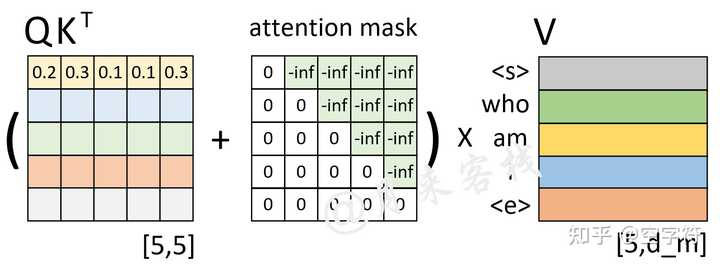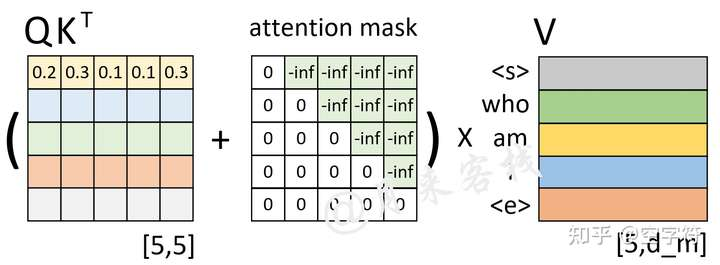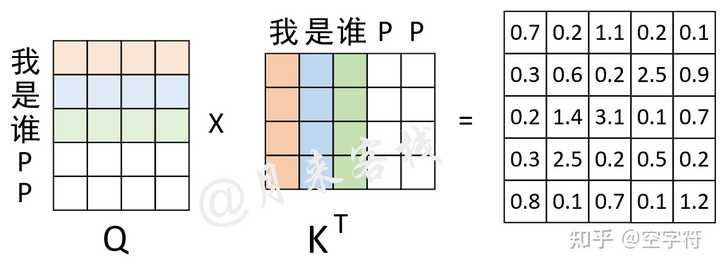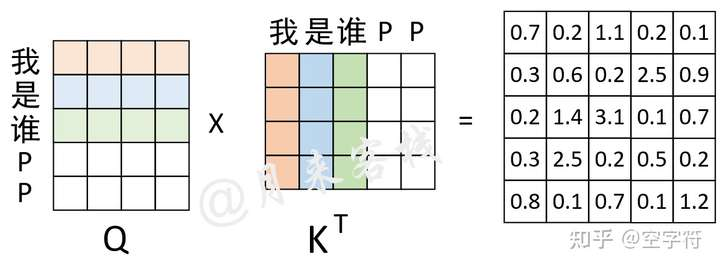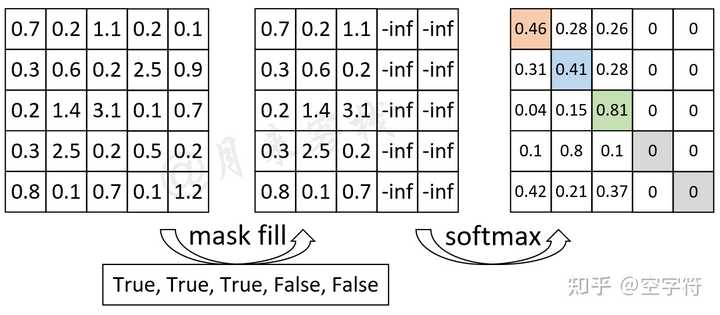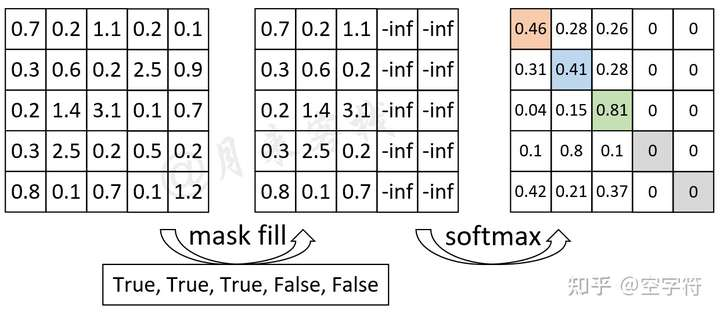2 实现多头注意力机制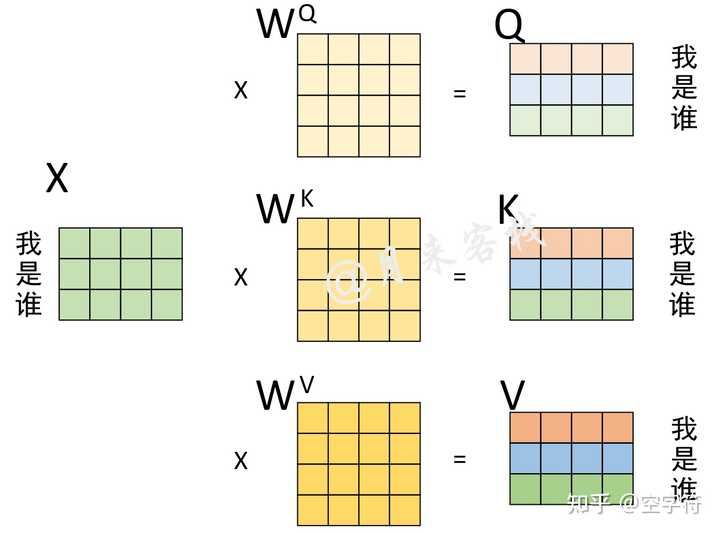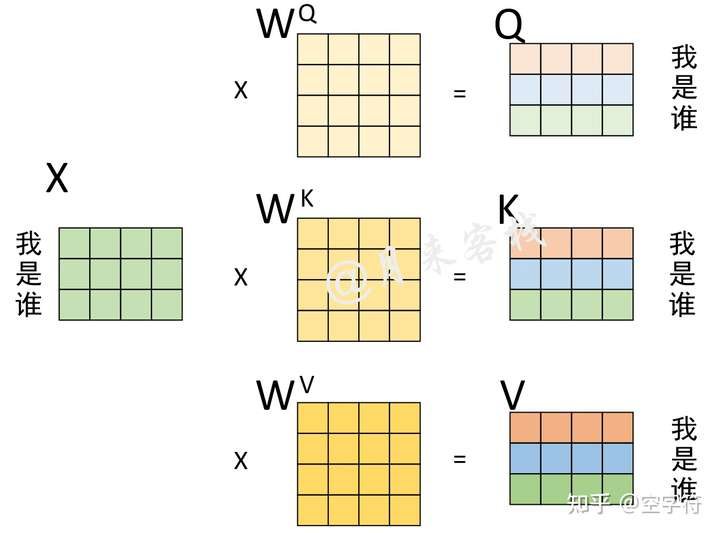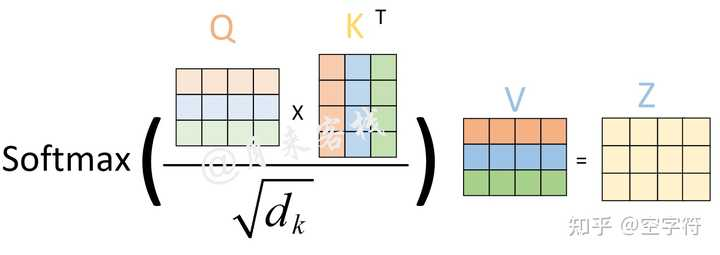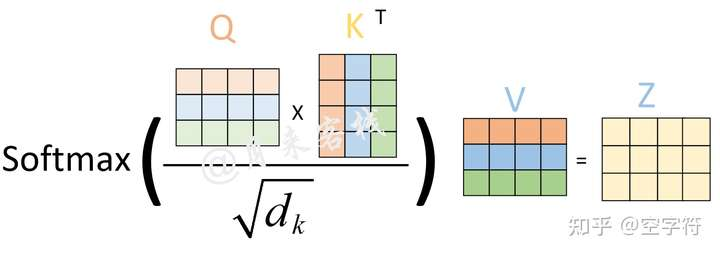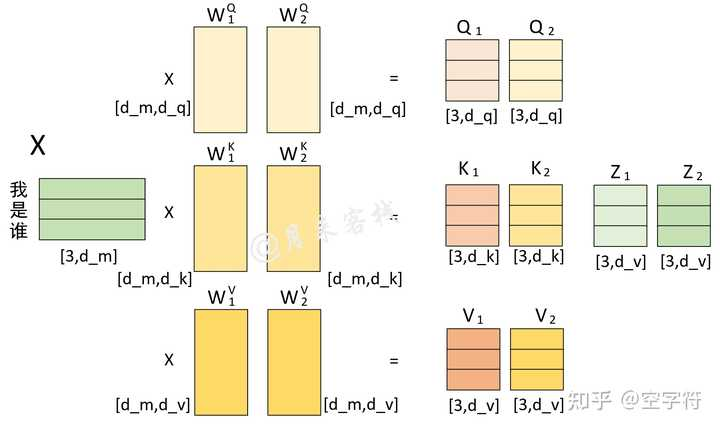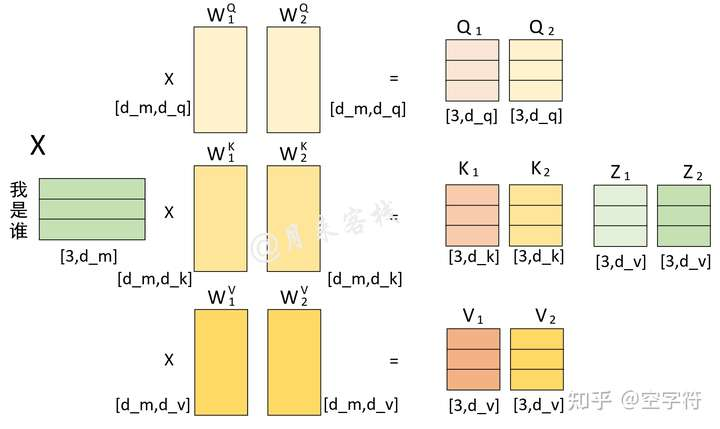def __init__(self, embed_dim, num_heads, dropout=0., bias=True):
"""
:param embed_dim:   词嵌入的维度，也就是前面的d_model参数，论文中的默认值为512
:param bias:        最后对多头的注意力（组合）输出进行线性变换时，是否使用偏置
"""
self.embed_dim = embed_dim  # 前面的d_model参数
self.dropout = dropout
# 上面的限制条件就是论文中的  d_k = d_v = d_model/n_head 条件
self.q_proj_weight = Parameter(torch.Tensor(embed_dim, embed_dim))  # embed_dim = kdim * num_heads
self.k_proj_weight = Parameter(torch.Tensor(embed_dim, embed_dim))  # W_k,  embed_dim = kdim * num_heads
self.v_proj_weight = Parameter(torch.Tensor(embed_dim, embed_dim))  # W_v,  embed_dim = vdim * num_heads
self.out_proj = nn.Linear(embed_dim, embed_dim, bias=bias)
# 最后将所有的Z组合起来的时候，也是一次性完成， embed_dim = vdim * num_heads

2.2 定义前向传播过程

"""
在论文中，编码时query, key, value 都是同一个输入，
解码时 输入的部分也都是同一个输入，
解码和编码交互时 key,value指的是 memory, query指的是tgt
:param query: # [tgt_len, batch_size, embed_dim], tgt_len 表示目标序列的长度
:param key:  #  [src_len, batch_size, embed_dim], src_len 表示源序列的长度
:param value: # [src_len, batch_size, embed_dim], src_len 表示源序列的长度
:return:
attn_output: [tgt_len, batch_size, embed_dim]
attn_output_weights: # [batch_size, tgt_len, src_len]
"""
self.dropout, self.out_proj.weight, self.out_proj.bias,
training=self.training,
q_proj_weight=self.q_proj_weight,
k_proj_weight=self.k_proj_weight,
v_proj_weight=self.v_proj_weight,

2.3 多头注意力计算过程

query,  # [tgt_len,batch_size, embed_dim]
key,  # [src_len, batch_size, embed_dim]
value,  # [src_len, batch_size, embed_dim]
dropout_p,
out_proj_weight, # [embed_dim = vdim * num_heads, embed_dim]
out_proj_bias,
training=True,
k_proj_weight=None,  # [embed_dim, kdim * num_heads]
v_proj_weight=None,  # [embed_dim, vdim * num_heads]
):
# 第一阶段： 计算得到Q、K、V
q = F.linear(query, q_proj_weight)
k = F.linear(key, k_proj_weight)
v = F.linear(value, v_proj_weight)

tgt_len, bsz, embed_dim = query.size()  # [tgt_len,batch_size, embed_dim]
src_len = key.size(0)
q = q * scaling  # [query_len,batch_size,kdim * num_heads]

if list(attn_mask.size()) != [1, query.size(0), key.size(0)]:
raise RuntimeError('The size of the 2D attn_mask is not correct.')
raise RuntimeError('The size of the 3D attn_mask is not correct.')

# 第三阶段： 计算得到注意力权重矩阵
attn_output_weights = torch.bmm(q, k.transpose(1, 2))

# 第四阶段： 进行相关掩码操作
attn_output_weights = attn_output_weights.view(bsz, num_heads, tgt_len, src_len)
# 变成 [batch_size, num_heads, tgt_len, src_len]的形状
# 扩展维度，从[batch_size,src_len]变成[batch_size,1,1,src_len]
attn_output_weights = attn_output_weights.view(bsz * num_heads, tgt_len,src_len)
# [batch_size * num_heads, tgt_len, src_len]

attn_output_weights = F.softmax(attn_output_weights, dim=-1)# [batch_size * num_heads, tgt_len, src_len]
attn_output_weights = F.dropout(attn_output_weights, p=dropout_p, training=training)
attn_output = torch.bmm(attn_output_weights, v)
# Z = [batch_size * num_heads, tgt_len, src_len]  x  [batch_size * num_heads,src_len,vdim]
# = # [batch_size * num_heads,tgt_len,vdim]

attn_output = attn_output.transpose(0, 1).contiguous().view(tgt_len, bsz, embed_dim)
# 先transpose成 [tgt_len, batch_size* num_heads ,kdim]
attn_output_weights = attn_output_weights.view(bsz, num_heads, tgt_len, src_len)

Z = F.linear(attn_output, out_proj_weight, out_proj_bias)
# 这里就是多个z  线性组合成Z  [tgt_len,batch_size,embed_dim]

2.4 示例代码

if __name__ == '__main__':
src_len = 5
batch_size = 2
dmodel = 32
src = torch.rand((src_len, batch_size, dmodel))  # shape: [src_len, batch_size, embed_dim]
[True, True, True, True, False]])  # shape: [src_len, src_len]

query的shape([tgt_len, batch_size, embed_dim]):torch.Size([5, 2, 32])
Q  的shape([tgt_len, batch_size,kdim * num_heads]):torch.Size([5, 2, 32])
----------------------------------------------------------------------
key 的shape([src_len,batch_size, embed_dim]):torch.Size([5, 2, 32])
K  的shape([src_len,batch_size,kdim * num_heads]):torch.Size([5, 2, 32])
----------------------------------------------------------------------
value的shape([src_len,batch_size, embed_dim]):torch.Size([5, 2, 32])
V  的shape([src_len,batch_size,vdim * num_heads]):torch.Size([5, 2, 32])
----------------------------------------------------------------------
多头线性变化后的形状为([tgt_len,batch_size,embed_dim]) torch.Size([5, 2, 32])

相关推荐

•0
•0
•3246收藏

• 66

关注
• 121

获赞
• 22

精选文章

• 工业瑕疵检测

• 小白学CV的专栏

• 768
• 925
• 769
• 2.1k
• 1.8k